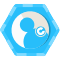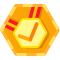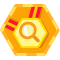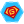## 1. BigDecimal

BigDecimal表示不可变的任意精度带符号十进制数。它由两部分组成：

• intVal - 未校正精度的整数,类型为`BigInteger`
• Scale - 一个32位整数，表示小数点右边的位数 例如，BigDecimal 3.14的未校正值为314，缩放为2。我们使用BigDecimal进行高精度算术运算。我们还将它用于需要控制比例和舍入行为的计算。如果你的计算是商业计算请务必使用计算精确的`BigDecimal`

## 2. 构造BigDecimal

``````     @Test
public void theValueMatches() {

BigDecimal bdFromString = new BigDecimal("0.12");
BigDecimal bdFromCharArray = new BigDecimal(new char[]{'3', '.', '1', '4', '1', '5'});
BigDecimal bdlFromInt = new BigDecimal(42);
BigDecimal bdFromLong = new BigDecimal(123412345678901L);
BigInteger bigInteger = BigInteger.probablePrime(100, new Random());
BigDecimal bdFromBigInteger = new BigDecimal(bigInteger);

assertEquals("0.12", bdFromString.toString());
assertEquals("3.1415", bdFromCharArray.toString());
assertEquals("42", bdlFromInt.toString());
assertEquals("123412345678901", bdFromLong.toString());
assertEquals(bigInteger.toString(), bdFromBigInteger.toString());

}

``````

``````@Test
public void whenBigDecimalCreatedFromDouble_thenValueMayNotMatch() {
BigDecimal bdFromDouble = new BigDecimal(0.1d);
assertNotEquals("0.1", bdFromDouble.toString());
}
``````

``````    @Test
public void whenBigDecimalCreatedUsingValueOf_thenValueMatches() {

BigDecimal bdFromDouble = BigDecimal.valueOf(0.1d);
BigDecimal  bigFromLong=BigDecimal.valueOf(1,1);

assertEquals("0.1", bdFromDouble.toString());
assertEquals("0.1", bigFromLong.toString());
}
``````

## 3. 常用API

abs() 绝对值，scale不变
subtract(BigDecimal augend) 减，scale为augend和原值scale的较大值
multiply(BigDecimal multiplicand) 乘，scale为augend和原值scale的和
divide(BigDecimal divisor) 除，原值/divisor，如果不能除尽会抛出异常，scale与原值一致
divide(BigDecimal divisor, int roundingMode) 除，指定舍入方式，scale与原值一致
divide(BigDecimal divisor, int scale, int roundingMode) 除，指定舍入方式和scale
remainder(BigDecimal divisor) 取余，scale与原值一致
divideAndRemainder(BigDecimal divisor) 除法运算后返回一个数组存放除尽和余数 如 `23/3` 返回 `{7,2}`
divideToIntegralValue(BigDecimal divisor) 除，只保留整数部分，但scale仍与原值一致
max(BigDecimal val) 较大值，返回原值与val中的较大值，与结果的scale一致
min(BigDecimal val) 较小值，与结果的scale一致
movePointLeft(int n) 小数点左移，scale为原值scale+n
movePointRight(int n) 小数点右移，scale为原值scale+n
negate() 取反，scale不变
pow(int n) 幂，原值^n，原值的n次幂
scaleByPowerOfTen(int n) 相当于小数点右移n位，原值*10^n

## 4. BigDecimal操作

BigDecimal上的操作就像其他Number类（Integer，Long，Double等）一样，BigDecimal提供算术和比较操作的操作。它还提供了缩放操作，舍入和格式转换的操作。它不会使算术运算符`+， - ，/，*`或逻辑运算符`>、< 、|、&` 过载。相反，我们使用`BigDecimal`相应的方法 - 加，减，乘，除和比较。并且`BigDecimal`具有提取各种属性的方法。

### 4.1 提取属性

``````@Test
public void whenGettingAttributes_thenExpectedResult() {
BigDecimal bd = new BigDecimal("-12345.6789");

assertEquals(9, bd.precision());
assertEquals(4, bd.scale());
assertEquals(-1, bd.signum());
}
``````

### 4.2 比较大小

``````@Test
public void whenComparingBigDecimals_thenExpectedResult() {
BigDecimal bd1 = new BigDecimal("1.0");
BigDecimal bd2 = new BigDecimal("1.00");
BigDecimal bd3 = new BigDecimal("2.0");

assertTrue(bd1.compareTo(bd3) < 0);
assertTrue(bd3.compareTo(bd1) > 0);
assertTrue(bd1.compareTo(bd2) == 0);
assertTrue(bd1.compareTo(bd3) <= 0);
assertTrue(bd1.compareTo(bd2) >= 0);
assertTrue(bd1.compareTo(bd3) != 0);
}
``````

``````@Test
public void whenEqualsCalled_thenSizeAndScaleMatched() {
BigDecimal bd1 = new BigDecimal("1.0");
BigDecimal bd2 = new BigDecimal("1.00");

assertFalse(bd1.equals(bd2));
}
``````

### 4.3 四则运算

BigDecimal 提供了以下四则运算的方法：

• subtract ——减法
• divide ——除法，有可能除不尽，必须显式声明保留小数位数避免抛出`ArithmeticException`异常
• multiply ——乘法
``````
@Test
public void whenPerformingArithmetic_thenExpectedResult() {
BigDecimal bd1 = new BigDecimal("4.0");
BigDecimal bd2 = new BigDecimal("2.0");

BigDecimal difference = bd1.subtract(bd2);
BigDecimal quotient = bd1.divide(bd2);
BigDecimal product = bd1.multiply(bd2);

assertTrue(sum.compareTo(new BigDecimal("6.0")) == 0);
assertTrue(difference.compareTo(new BigDecimal("2.0")) == 0);
assertTrue(quotient.compareTo(new BigDecimal("2.0")) == 0);
assertTrue(product.compareTo(new BigDecimal("8.0")) == 0);
}
``````

### 4.4 四舍五入

• RoundingMode.UP：以小数位为原点 是正数取右边，负数取左边
• RoundingMode.DOWN：以小数位为原点 也就是正数取左边，负数取右边
• RoundingMode.FLOOR：取左边最近的正数
• RoundingMode.CEILING：取右边最近的整数
• RoundingMode.HALF_DOWN:五舍六入，负数先取绝对值再五舍六入再负数
• RoundingMode.HALF_UP:四舍五入，负数原理同上
• RoundingMode.HALF_EVEN:这个比较绕，整数位若是奇数则四舍五入，若是偶数则五舍六入
• RoundingMode.ROUND_UNNECESSARY：不需要取整，如果存在小数位，就抛ArithmeticException 异常

## 5. 格式化

### 5.1 NumberFormat

• getInstance(Locale)、getNumberInstance(Locale)。返回指定语言环境的通用数值格式。
• NumberFormat.getCurrencyInstance(Locale)。 返回指定语言环境的货币格式。
• NumberFormat.getPercentInstance(Locale)。 返回指定语言环境的百分比格式。
• NumberFormat.getIntegerInstance(Locale)。 返回指定语言环境的整数数值格式。
• NumberFormat.setMinimumIntegerDigits(int)。设置数的整数部分所允许的最小位数。
• NumberFormat.setMaximumIntegerDigits(int)。设置数的整数部分所允许的最大位数。
• NumberFormat.setMinimumFractionDigits(int)。设置最少小数点位数，不足的位数以0补位，超出的话按实际位数输出。
• NumberFormat.setMaximumFractionDigits(int)。设置最多保留小数位数，不足不补0。

### 5.2 DecimalFormat

`DecimalFormat`除了能代理上面的`NumberFormat`以外，还提供了基于`pattern`字符串的格式化风格，有点类似格式化时间一样。我们来看看`pattern`的规则:

• “0”——表示一位数值，如没有，显示0。如“0000.0000”，整数位或小数位>4，按实际输出，<4整数位前面补0小数位后面补0，凑足4位。

• “#”——表示任意位数的整数。如没有，则不显示。在小数点位使用，只表示一位小数，超出部分四舍五入。如：“#”：无小数，小数部分四舍五入。“.#”：整数部分不变，一位小数，四舍五入。“.##”：整数部分不变，二位小数，四舍五入。

• “.”——表示小数点。注意一个pattern中只能出现一次，超过一次将格式化异常。

• “，”——与模式“0”一起使用，表示逗号。注意一定不能在小数点后用，否则格式化异常。

## 总结

`关注公众号：码农小胖哥 获取更多资讯`### 码农小胖哥andyqian
2019/07/18
83
0
Java BigDecimal详解

JackieYeah
2014/03/05
1.9K
8
Binomial Coefficient（二项式系数）

In mathematics, any of the positive integers that occurs as a coefficient in the binomial theorem is a binomial coefficient. Commonly, a binomial coefficient is indexed by a pai......

honeymose
2018/12/30
40
0

leqianlong
2014/01/22
417
0
java.math.BigDecimal记录

1.引言 借用《Effactive Java》这本书中的话，float和double类型的主要设计目标是为了科学计算和工程计算。他们执行二进制浮点运算，这是为了在广域数值范围上提供较为精确的快速近似计算而精...

2018/05/30
77
0

npm 发布webpack插件 webpack-html-cdn-plugin

31分钟前
55
0
java基础(16)递归

35分钟前
35
0
REST接口设计规范总结

URI格式规范 URI中尽量使用连字符”-“代替下划线”_”的使用 URI中统一使用小写字母 URI中不要包含文件(脚本)的扩展名 URI命名规范 文档(Document)类型的资源用名词(短语)单数命名 集合(Co...

Treize

57
0
CentOS-配置YUM源加速(阿里云、Nexus3)

-自由人生-

80
0

javail

55
0